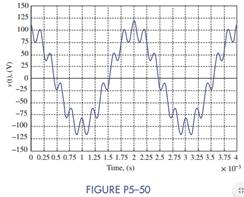Create an Account

Home / Questions / Figure P5–50 is the result of the sum of a fundamental and one of its harmonics (an integer...

Figure P5–50 is the result of the sum of a fundamental and one of its harmonics (an integer...

Figure P5–50 is the result of the sum of a fundamental and one of its harmonics (an integer multiple of the fundamental). Find VMAX, VMIN, Vp, Vpp, Vavg, Vrms, and T0 for the waveform.Jul 28 2020 View more View LessSubscribe To Get Solution